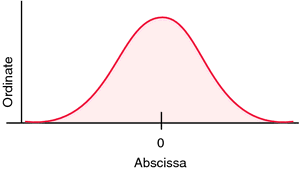abscissa

(redirected from Abscissæ)
Also found in: Dictionary, Thesaurus, Encyclopedia.

abscissa

[ab-sis´ah]
the horizontal line in a graph along which are plotted the units of one of the variables considered in the study, as time in a time-temperature study. The other line is called the ordinate.Axes of graph showing normal distribution curve.

ab·scis·sa

(ab-sis'ă),
In a plane cartesian coordinate system, the horizontal axis (x). Compare: ordinate.
[L. ab-scindo, pp. -scissus, to cut away from]

abscissa

/ab·scis·sa/ (ab-sis´ah) the horizontal line in a graph along which are plotted the units of one of the factors considered in the study. Symbol .

abscissa

[absis′ə]
Etymology: L, ab, away; scindere, to cut
a point on a horizontal Cartesian coordinate plane measured from the y-axis (or vertical axis) running perpendicular to the plane, or the x-axis.

ab·scis·sa

(ab-sis'ă)
In a plane cartesian coordinate system, the horizontal axis (x).
[L. ab-scindo, pp. -scissus, to cut away from]

abscissa

the horizontal line in a graph along which are plotted the units of one of the factors considered in the study, as time in a time-temperature study. The other line is called the ordinate.
Site: Follow: Share:
Open / Close